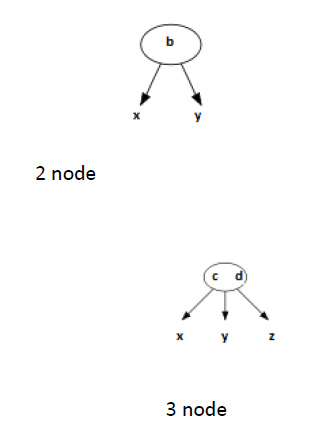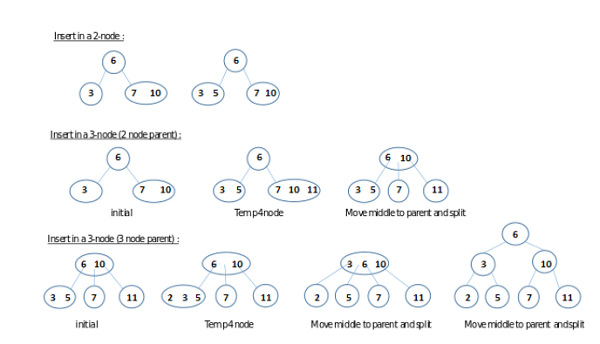# 2-3 Trees (Search and Insert) in C/C++?

A 2-3 tree is defined as a tree data structure, where every node with children (internal node) has either two children (2-node) as well as one data element or three children (3-nodes) as well as two data elements.Definitions

We call that an internal node is a 2-node if it has one data element and two children.

We call that an internal node is a 3-node if it has two data elements and three children.

We call that T is a 2-3 tree if and only if one of the following statements satisf −

• T is vacant or empty. In other words, T contains no have any nodes.

• T is a 2-node equipped with data element a. If T has left child L and right child R, then we conclude

• L and R are treated as non-empty 2-3 trees of the same height;

• a is higher than each element in L; and

• a is smaller than or equal to each data element in R.

• T is a 3-node with data elements a and b, where a is less than b. If T has left child L, middle child M, and right child R, then we conclude

• L, M, and R are treated as non-empty 2-3 trees of equal height;

• a is higher than each data element in L and smaller than or equal to each data element in M; and

• b is higher than each data element in M and smaller than or equal to each data element in R.

## Properties

• Every internal node is treated as a 2-node or a 3-node.

• All leaves are located at the same level.

• All data is maintained or kept in sorted order.

## Operations

### Searching

Searching for an item in a 2-3 tree is same as searching for an item in a binary search tree. Since the data elements in each node are treated as ordered, a search function will be directed to the correct subtree and eventually to the correct node which consists of the item.

• Let T be treated as a 2-3 tree and d be the data element we want to find. If T is empty, then d is not in T and we are performed.

• Let r be treated as the root of T.

• Let r is a leaf. If d is not in r, as a result d is not in T. Otherwise, d is in T. In particular, d can be found at a leaf node. We need no further steps and we are performed.

• Suppose r is treated as a 2-node with left child L and right child R. Let e be treated as the data element in r.

There are three cases −

• If d is equal to e, then we've found d in T and we are performed.

• If d is less than e, then set T to L, which by definition is a 2-3 tree, and return back to step 2.

• If d is greater e, then set T to R and return back to step 2.

• Let r is a 3-node with left child L, middle child M, and right child R. Let a and b be treated as the two data elements of r, where a<b. There are four cases −

• If d is equal to a or b, then d is in T and we are performed.

• If d is less than a, then set T to L and return back to step 2.

• If ais less than d and d is less than b, then set T to M and return back to step 2.

• If d is greater than b, then set T to R and return go back to step 2.

### Insertion

Insertion performs by searching for the proper location of the key and appending or adding it there. If the node becomes a 4-node then the node is divided into two 2-nodes and the middle key is moved up to the parent. The parent may then become a 4-node, in which case it is divided too, propagating a key to its own parent. This process repeats until and unless we reach a parent which is a 2-node and does not need to be divided, or when we reach the root, in which case we implement the propagated element to create a new root which is a 2-node. With the help of this algorithm, the number of operations to perform is proportional to the height of the tree, hence logarithmic because the tree is balanced perfectly. The process ensures that its result is treated as a 2-3 tree:in particular, all leaves remain at the same depth.

The diagram below explains or illustrates possible cases of this process.Insertion of a number in a 2-3 tree for 3 possible cases.